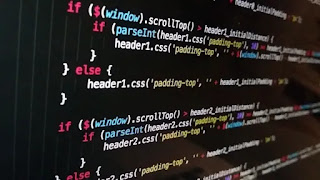# Except | Python Try Except - codebugfree

In this section we learn the python Error handling. Error handling is handle the error in python. It is also known as Python Try Except. Try except handling the user input error value. Detect the user input error value and rise the error.Python Except and try for error handling

Try Except save from the program crash. If user input alphabet to integer than the integers cannot handle the alphabet than the program is crash. So when programmer use try except to detect the problem and give output the user.

Mainly benefits of try except is make program with out bug and crash able. Try Except is a python built in function. Module does not requirement to use try except.

Using the try except also rise the particular error. Using the rise only specific one error handling. Other error find than program crash.

Programmer can alo use try for program run raise for specific error and other error handling with except.

Now we see the code with using try except and rise the error. Also describe the code.

``````try:
first_number = int(input("Enter your number: "))
multiply_5 = 1
for i in range(first_number):
multiply_5 = multiply_5 * first_number
print(multiply_5)
except Exception as e:
e = "inval;id activity"
print(e)``````

Here use try first inside the try input the number starting the for loop with rage the user input first number. print the multiply. Last using the except to print if any user input error.

INOUTPUT

``````5
25
125
625
3125``````

It is a out with given input any error value. Now we given input error value and see the error handling.

INOUTPUT

``````Enter your number: codebugfree
inval input activity``````

Now see the another code

``````try:
User_number = int(input("Enter any number: "))
if User_number%2 == 0:
else:
print("You input a odd number")
except Exception as e:
e = " please input a number"
print("e")``````

Inside the try block get input from user and using if else condition with user input number is divide by 2 or not. If divided by 2 and remander is o than print if statement other wise print the else statement.

INOUTPUT

``````Enter any number: 45
You input a odd number``````

INOUTPUT with given error input

``````Enter any number: codebugfree